18min

# SiClone - UV mapping

## Applying Materials

Apply materials to the reference objects and not SiClone. Think of SiClone as a container of referenced 3D objects (array), and splines and shapes (sweeps).

## Warning - UVW Modifiers!

If you apply a UVW modifier to the spline, it won’t work and may crash 3ds Max. If it’s a closed spline 3ds Max converts it automatically to and edit mesh!

### 01. Apply materials to the splines and shapes

Apply the material to the splines referenced by SiClone and never directly to the SiClone model itself.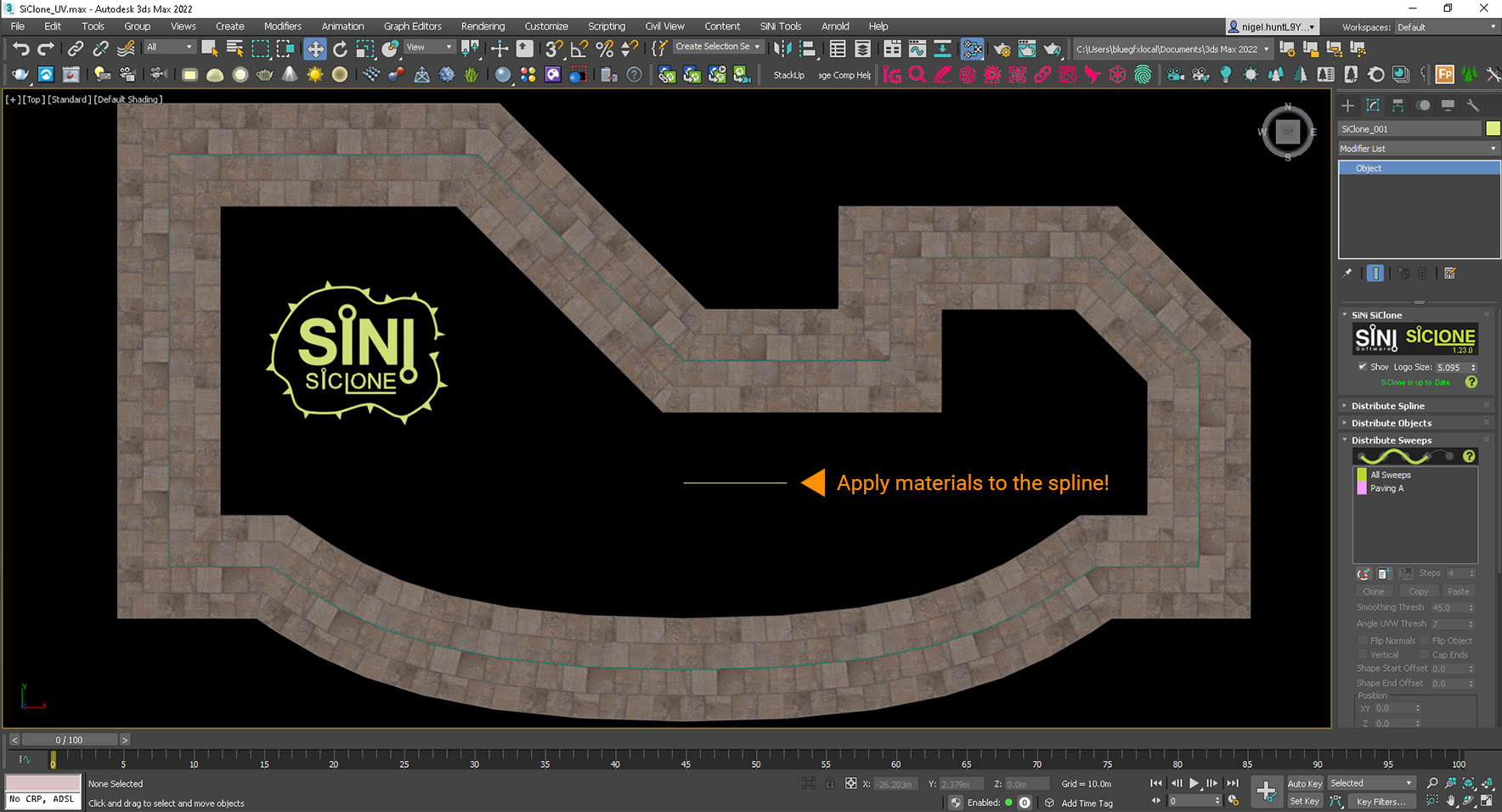Add materials to the spline and not SiClone.

You can apply any materials to SiClone. We have used V-Ray 5 material shaders, downloaded from the V-Ray Material Library Browser.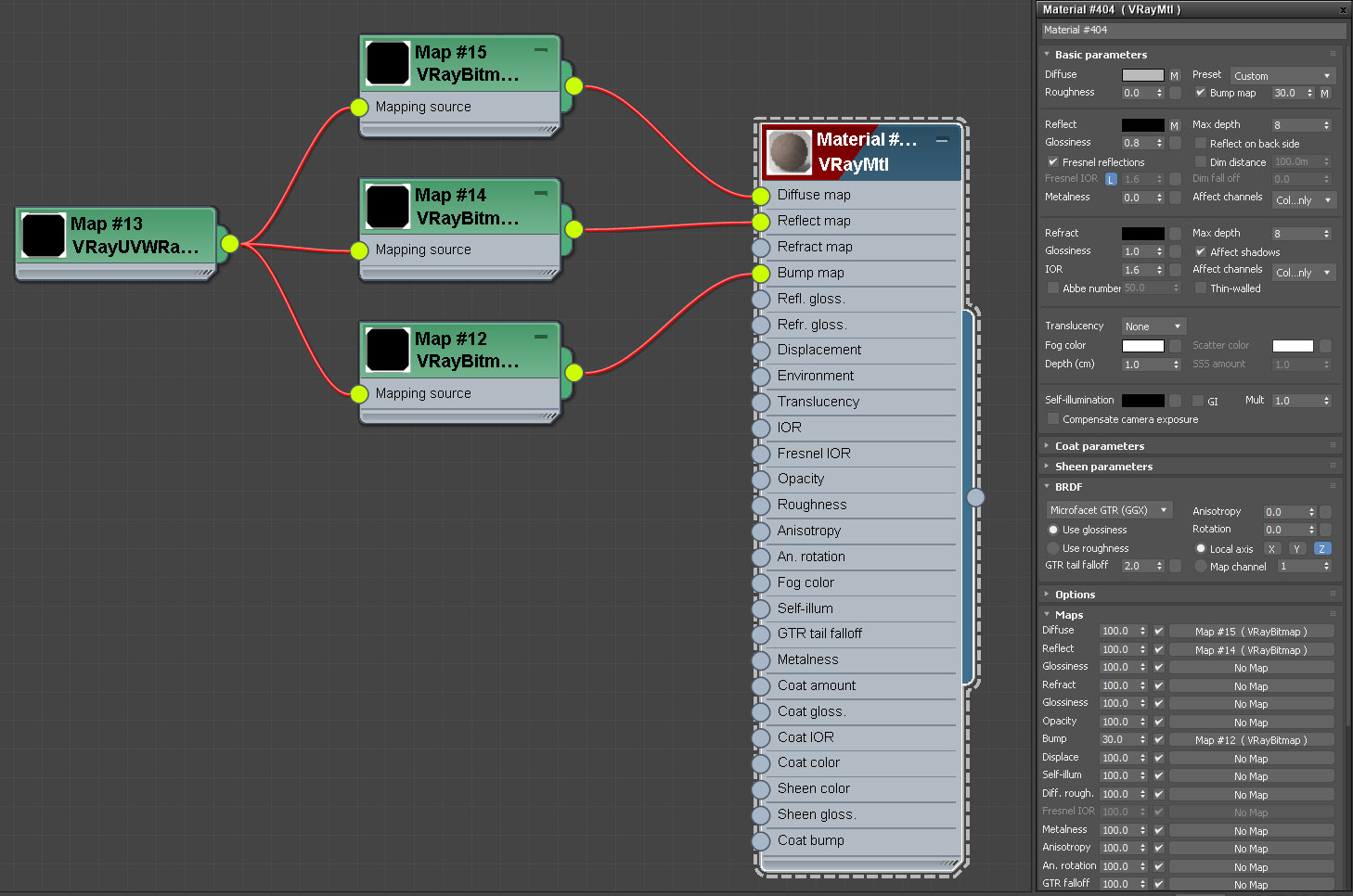V-Ray material applied to the spline.

In the SiClone Distribute Sweeps rollup add the textured splines and shapes you wish to include.

Then in the 3ds Max materials editor, adjusting the UV properties.

In the V-Ray UI image below we adjust the real-world scale. The U equals the spline width and the V equals the length. Because SiClone is parametric, and the length is able to change, we use SiClone V tiling to determine the V length. See below.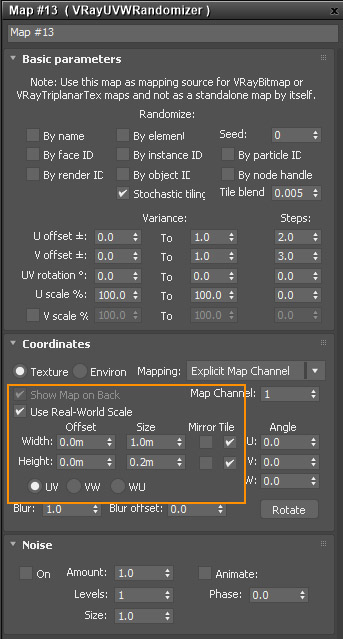Using Real-World scale set to 1 (U) by 0.2 (V).

The below image shows the spline in SiClone with its parametric tiled textures. This means the tiling will always follow the spline direction, even when you modify the spline path.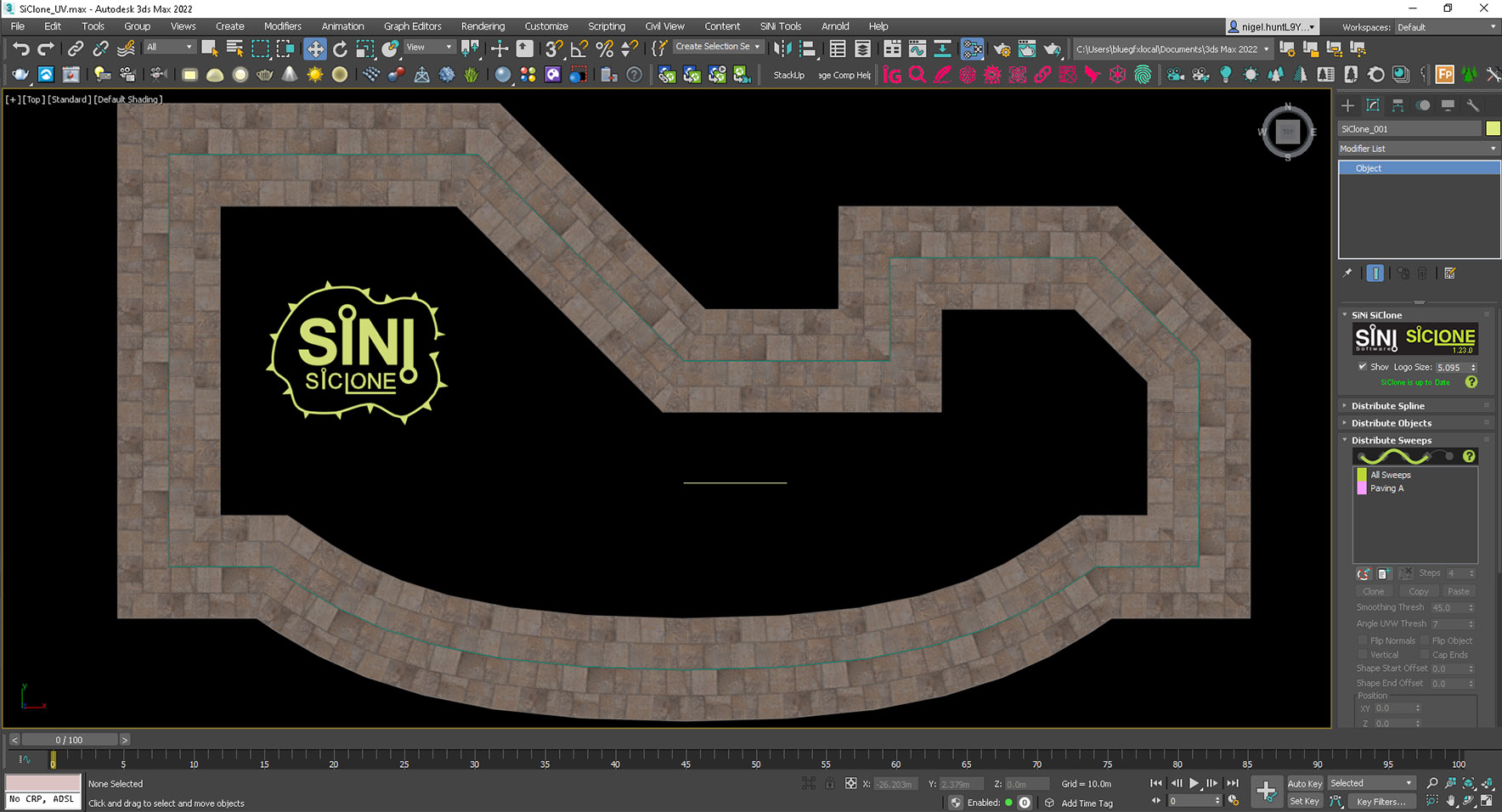The spline added to SiClone - Distribute Sweeps.

### SiClone V Tiling - (SVT)

At present SiClone uses a predetermined tiling formula to calculate the length (V). This is set at 25 units translated into different system/scene units as follows. This is necessary to allow for parametric adjustments of the SiClone model.

 Units SiClone (V) code Translated units Millimetres 25 25000 mm Centimetres 25 2500 cm Metres 25 25 m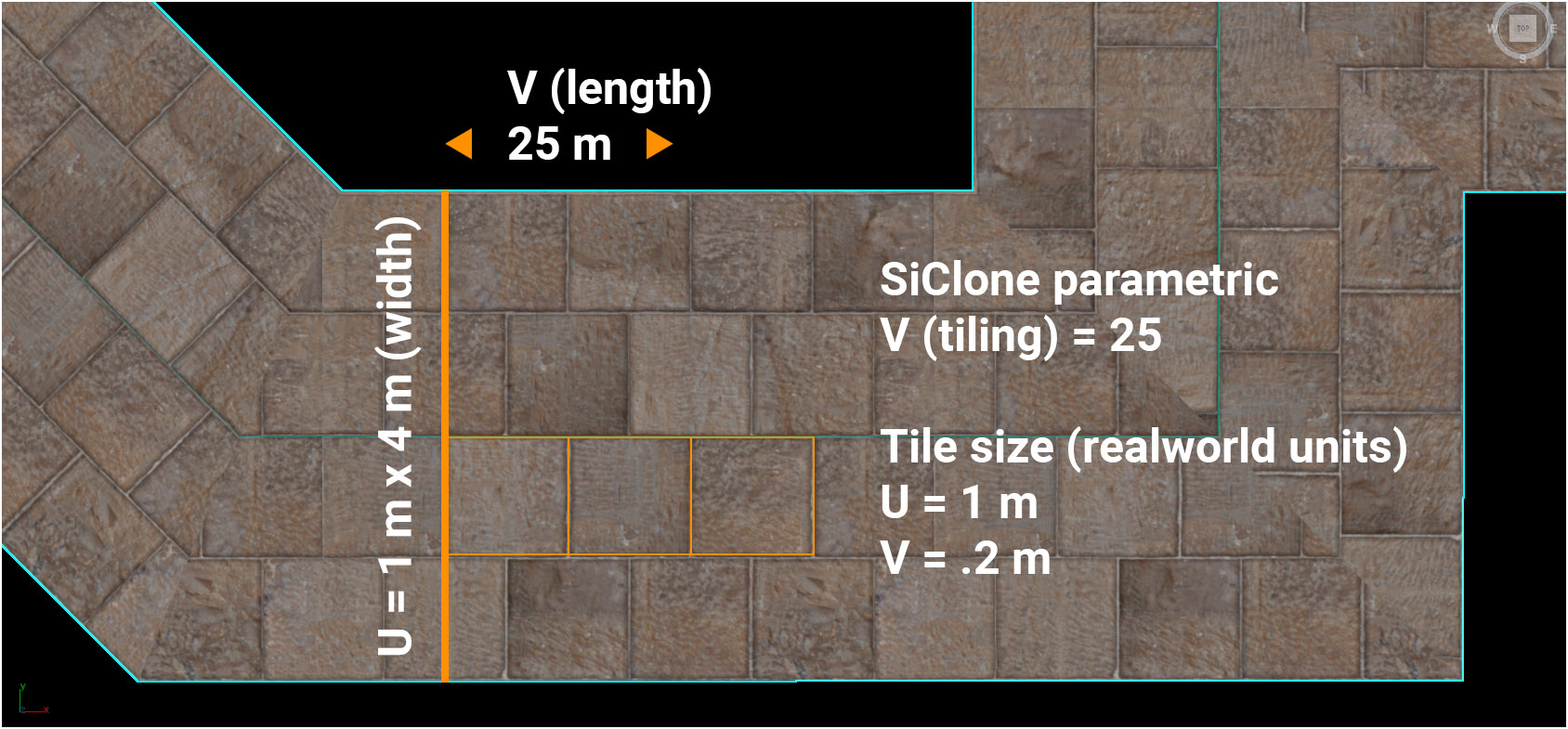Maths to calculate the SiClone tiling.

As an example, the image above demonstrates how the material uses a V-Ray real-world material set to a 1 m wide tile (U).

If we want a real-world length of 1 m then the formula is; 1 m x 1 m UV tiles. SVT = 25 m. Tile = 1 m (The bitmap has 4 (U) x 5 (V) tiles). 5 (V) normal units (25 / 5 (V) bitmap tiles). 0.2 (V) real-world input (0.2 m x 5 (bitmap tiles) = 1 m tile size. 1 m tile x 5 = 5 m x 5 = 25 SVT.

### SiClone V Tiling explained

The image below shows a 25 m long spline with a checker pattern applied to the reference spline. It demonstrates how to create a rectangular checker.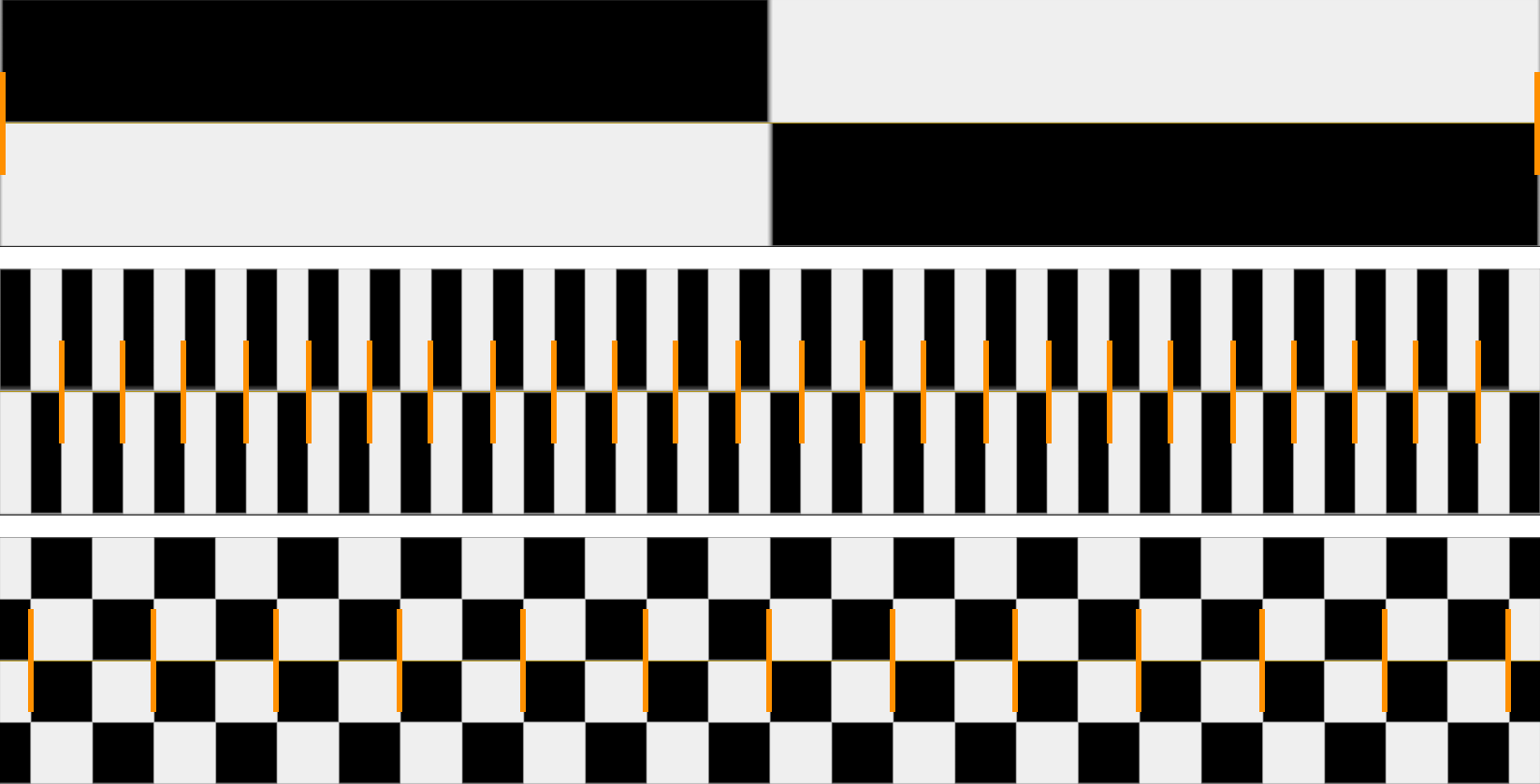UV SiClone V tiling comparison.

UV Setout tiling (Not real-world)

• Top row - 25 m SiClone V tiling set to 1.
• Middle row - 25 m SiClone V tiling set to 25. 2 checker tiles per tile (V).
• Bottom row - 25 m SiClone V tiling set to 12.5 to double checker tiles in (V).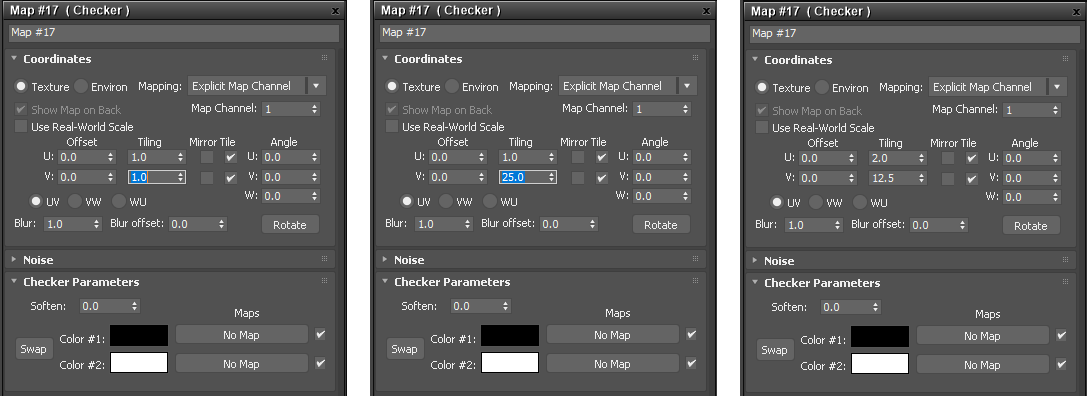UV inputs for the checker setups above.

From left to right UV tiling.

• Left - 25 m SiClone V tiling set to 1.
• Middle - 25 m SiClone V tiling set to 25.
• Right - 25 m SiClone V tiling set to 12.5. U is set to 2.

Using real-world UV mapping

To match the same results using real-world tiling we set the size as follows.

From left to right UV tiling.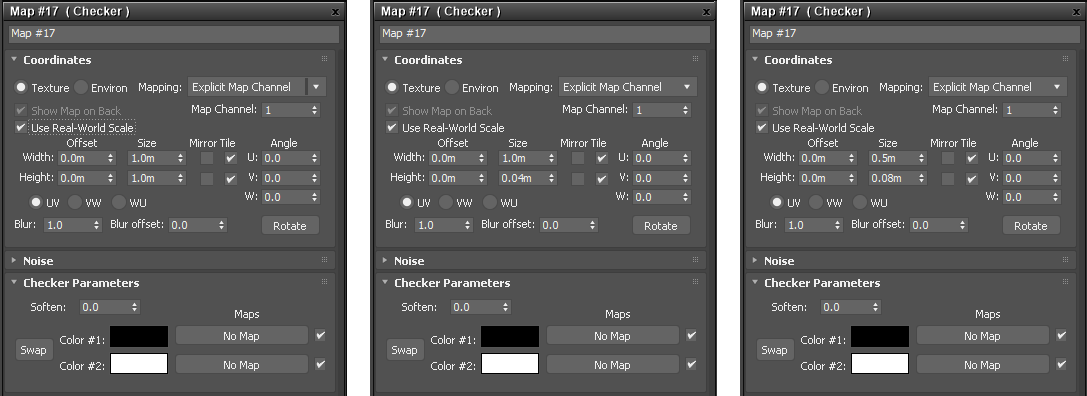UV inputs for the checker setups above.
• Left - 25 m SiClone V tiling set to 1.
• Middle - 25 m SiClone V tiling set to 0.04 m. (4 cm x 25 = 1 m)
• Right - 25 m SiClone V tiling set to 0.08 m. U is set to 0.5 m. (8 cm x 25 = 2 m or 2 x 1 m tiles).

We plan to update the formula to expose a user SVT value for custom accuracy rather than the preset 25.

## Tip - Use a scale reference object!

Its a good idea to place a reference 3D object over the SiClone sweep to act as a scale guide, as seen in the following image samples.100 cm SiClone V tiling - 50 cm stone tiles.

100 x 100 cm UV tiles (4 x 4 stone pavers bitmap). SVT = 2500 cm. 25 (V) normal tiling input. 4 cm (V) real-world input (4 cm x 25 = 100 cm tile).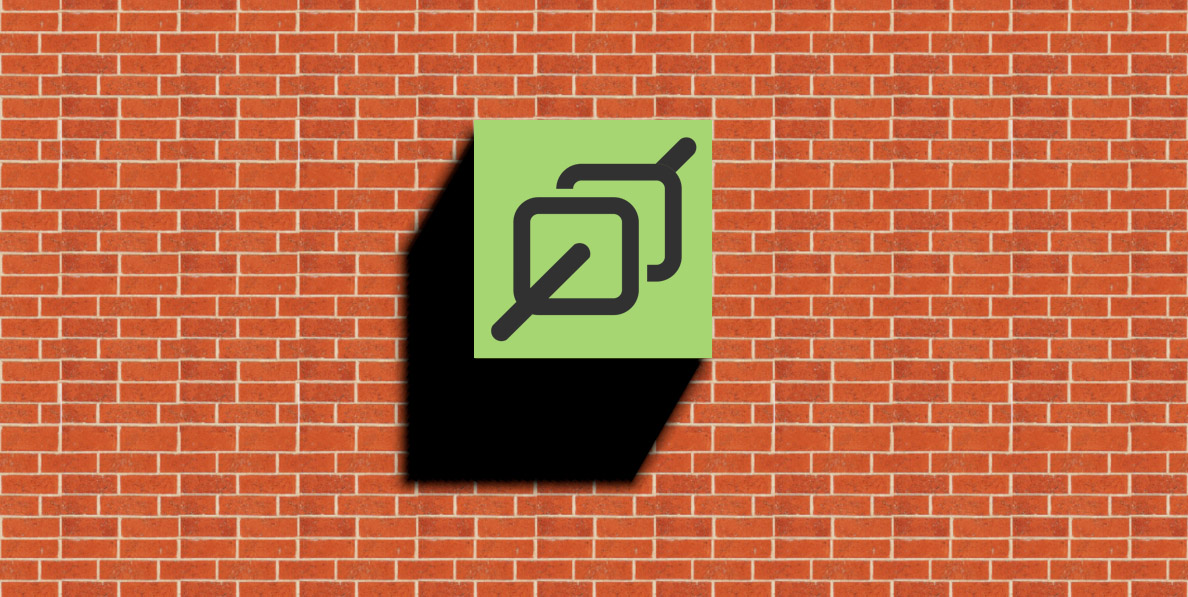90 cm SiClone V tiling - 22.5 cm bricks and motar.

21.5 cm UV bricks and mortar tiles (4 x 4 brick tileable bitmap - brick+motar length = 22.5 cm x 4 = 90 cm tile). SVT = 2500 cm. 27.7 (V) normal tiling input. 3.6 cm (V) real-world input (3.6 cm x 25 = 90 cm tile).

﻿

﻿

﻿

﻿

Updated 30 May 2021